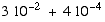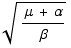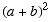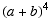Statistics Math Diagnostic

Please do this without a calculator, computer, or other assistance.

1. Find 23% of 50.

2. Find.

3. Findwhere μ = 16, α = 9, and β=1.

4. Expand.

5. Expand.  Explain your method.

6. What's the last math course you took, and when was that?

Converted by Mathematica  (August 28, 2003)